# SUM Function In Excel To Add Numbers Examples

In this tutorial, learn how to use the SUM function in Excel to calculate the total of numbers. Find the total for the numbers placed in a sequence of range or non-sequence cells.

Find the total of two or more numbers using SUM() with the step-by-step examples given below.

## What is the SUM Function in Excel

A `SUM()` is the mathematical function comes under category Math/Trig of Excel. It can be used in an Excel sheet to calculate the total of two or more numbers.

You can calculate the total of all the numbers using the SUM function. Use the methods and examples given below to add numbers of your excel sheet.

## Syntax of SUM Function in Excel

The syntax of SUM function contains multiple arguments as the numbers to add. It requires at least two numbers to perform addition.

If you want to put numbers directly in the SUM function. Use the syntax of `SUM()` given below.

However, if you want to add the numbers using cell names of the numbers. You have to use the syntax given below.

## Description of Arguments Passing to SUM Function

There are two arguments to pass in the SUM() function. The description of these arguments is given below.

Parameter Name Description
number_n These are the numbers which you have to use and calculate the total.
cell_n These are the names of the cells that contain the numbers. You can select as many cells as you want.

Now, if you understand the syntax of `SUM()` a function and the arguments. Let’s start using it in the various examples given below.

## Calculate Addition of Two or more Numbers in Range in MS Excel

You can calculate the total of the numbers in Range. A range is a sequence of cells in columns or rows in Excel Sheet. If you want to learn more about the range, please read our post on cell range in Excel with operations.

Now, to calculate the sum of two or more numbers arrange in the sequence of range in Excel. Follow the step-by-step guide given below with screenshots.

1. Decide Numbers in range to calculate SUM
First of all, decide on the number which you have to use and calculate the sum. Let’s take two numbers in Excel placed sequentially in column B. The range is from B2 to B3 to use and find the total.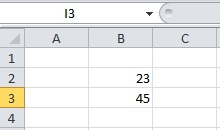The above image showing the two numbers in the range from B2 to B3.

2. Put `=SUM` in the Cell Where You Calculate Total
Suppose, you have to find the total in the cell B4. You have to place the code `=SUM` in the cell B4. This is the starting part of calculating the total of the numbers.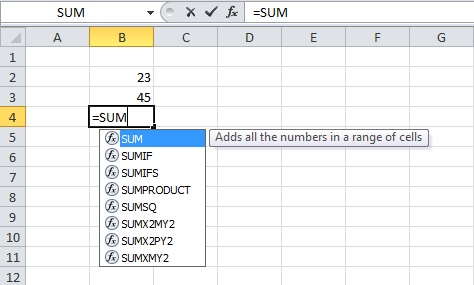You can check the above image to find how to place the code in the B4 cell.

3. Put start Bracket After SUM Function
A start bracket is to place after the code you have entered in the previous step. When you place the start bracket, you will see the syntax of SUM function as given above in this post.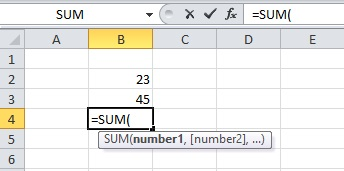The above image showing the syntax of the function comes after you put the start bracket.

4. Select All the Numbers in Range
Now, you have to just select the numbers to calculated total and placed in range. When you select the cells in the range contains the numbers. The range automatically gets entered inside the function as an argument.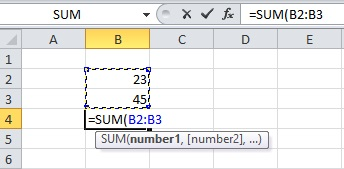The above example showing the range B2:B3 inside the function. This shows that you have selected the cell in the range from B2 to B3.

5. Put Close Bracket After the Range Name
After you got your range cells in the function, you have to put the close bracket. The close bracket completes the SUM function as given in the syntax above in this post.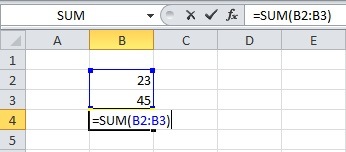The above examples showing the function which contains the cell range. It also contains the start bracket and the close bracket.

6. Press Enter to Get Total
Now, the final step in the calculation of the total is to press the enter button. You will get the final total of the cells contains the numbers.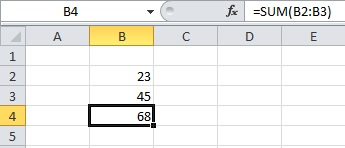The final total of the numbers will appear as the image given above.

You can find the total of your numbers placed sequentially in range. Change the numbers in the given example as per your requirement.

What will you do if you have numbers placed in a non-sequential manner? Well, you can use the same SUM function and use the steps given in the below section.

So, let’s start learning the method to find the total for non-sequential range cell in Excel.

## Add Numbers Placed in Non-sequential Cells in Excel

To explain how to calculate the total for the numbers placed in a non-sequential manner. Let’s take the example of numbers placed in the Excel sheet as shown in the image below.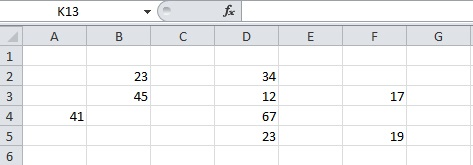There are many numbers in the above image example. Few are arranged in range while few are singly placed in Excel. You have to follow the step-by-step process given below.

1. Place `=SUM` at the cell where you calculate Total
Choose the cell where you want to calculate the total of all the numbers. After you choose the cell, place the code `=SUM` in the cell where you have to find the total.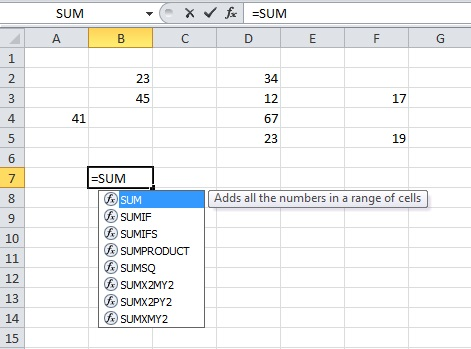The image given above shows that you are going to calculate the total in the cell B7. The placed code is showing the B7 cell.

2. Put Start Bracket after SUM Function
To start the function, you have to place the start bracket after the code of the above step. When you place the start bracket, a syntax of the SUM function starts appearing as shown in the image below.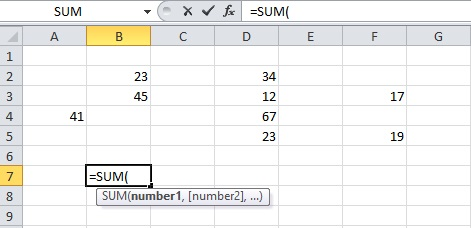The above showing that, the sum function requires arguments as numbers. However, the syntax is given in this page showing that you can also place cell names as arguments.

3. Press and Hold keyboard ‘CTRL’ button and select all Cells to contain numbers
After you place the start bracket in the SUM function. You have to press and hold the ‘CTRL’ button of your keyboard. Now, click all the cells contains the number while holding the ‘CTRL’ key. This will select all the cells contains the numbers to calculate the total.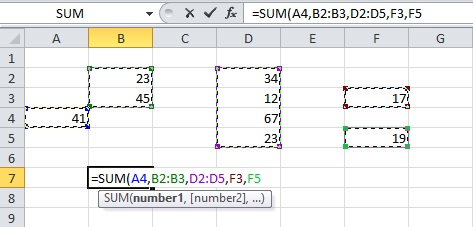The above image showing the selected cells contains numbers. The numbers are not in any sequence and placed in random cells.

4. Put Close Bracket After Selected Cell Names
The cell names are selected inside the function. You have to now put the close bracket to complete the function.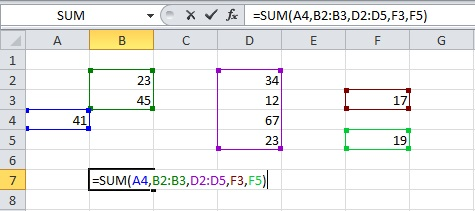The close bracket is showing in the above image.

5. Press Enter to Get Total
This is the final step where you have to just press the enter key of the keyboard. The total of the selected numbers starts showing in the cell where you want to calculate the total.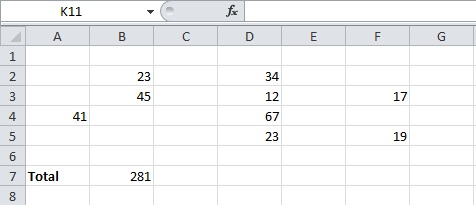The above image showing 281 as the total of numbers using the SUM Function In Excel.

You can as many numbers in the random cells of Excel as you want. After that, you can find the total of all the numbers using the SUM Function In Excel.

You may also like to read

Hope, you like this post of using SUM Function In Excel To Add Numbers. If you have any query regarding the tutorial, please comment below.

References

Also tell me, what other methods you are using with SUM Function.